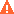HOME COURSES PREVIEW REVIEW ABOUT
 TOLL-FREE INFO & ORDERING: M-F: 9am-5pm (PST): (877) RAPID-10
 QUICK TOUR: Quick Movie - How to Learn in 24 Hours
 QUICK SEARCH: Keywords:
 Rapid Learning Member Area:Note: If you are a legacy user of chemistry24 members, please request a new login access to the premium server with your full name and old login email via vip@rapidlearningcenter.com
 RAPID COURSE CATALOG: Chemistry in 24 Hours
CHEMISTRY TIPS WEEKLY:

Are you taking a chemistry course or preparing for a chemistry exam? If you are learning chemistry now, the Chemistry Tips Weekly is for you--it is a one minute learning each week to the chemistry mastery, free for all students. The chemistry tips will include the follwing topics

• Chemistry survival basics
• Top ten confusions in chemistry
• How to ace chemistry exams
• Problem-solving tips in chemisrty
• Plus much more...
Enter your name and email address below and click "Subscribe" to have free Chemistry tips delivered to your inbox weekly.

 OTHER RELATED SITES: ChemistrySurival.com BiologySurvival.com Biology24.com Physics24.com Mathematics24.com RapidLearningCenter.com
 Note: For course links to launch, disable popup blockers or hold the ctrl key while clicking the link.Bonus - Free Coaching for 3 Months :  Need help on your homework or exam questions? Get stuck on chemistry problems? Let Dr. Wayne and his team of faculty help you personally. Order one of our 24-hour rapid chemistry courses today and you will receive 3-month chemistry coaching (\$297 value - free). Limited to first 250 students only. Grab yours today!

The Mole

 Topic Review on "Title": Atoms and molecules are so tiny that they need to be counted in very large quantities in order to result in a “workable” number.  The mole The mole (abbreviation: mol) is used to count particles.  1 mole of anything is 6.02 ´ 1023 of that thing.  Molar mass We cannot count out a mole of particles in the lab, therefore a connection between moles and mass is needed.  The molar mass is the mass (in grams) for 1 mole of the particle.  Molar mass (also often called Molecular mass, formula weight, etc.) is found by adding the atomic masses (multiplied by the number of that atom) for each atom in the molecule.  Molar mass can then be used in dimensional analysis conversions as the equality between grams and moles.  The molar mass value always goes with the “grams” in such conversions. Percent Composition “Percent” is part / whole ´ 100.  The “part” is the mass of an individual element while the “whole” is the mass of the whole molecule or sample.  These calculations can be done with lab data—grams of both the individual element and the whole sample—or with chemical formulas.  In the case of chemical formulas, the molar mass is used in the calculations. Empirical Formula The empirical formula is the lowest possible ratio of atoms in a molecule.  The ratio of atoms is the same as the ratio of moles of atoms.  The mass of each element is converted to moles and then all the moles are divided by the smallest to reach the lowest possible whole number ratio.  That ratio is used as the subscripts in writing the empirical formula. Molecular Formula The molecular formula is the actual ratio of atoms in a molecule.  The molar mass of the empirical formula is compared to the given molar mass of the molecular formula.  The comparison results in a factor that is used to multiply the subscripts of the empirical formula to reach the subscripts of the molecular formula.

Rapid Study Kit for "Title":
 Flash Movie Flash Game Flash Card Core Concept Tutorial Problem Solving Drill Review Cheat Sheet"Title" Tutorial Summary : Atoms and molecules are too small to be counted individually, so a counting unit of “mole” is used.  Molar masses are used to convert between mass and moles of atoms or molecules.  There are several ways to express the ratio of moles of atoms in a sample.  The tutorial introduces percent composition, empirical formula and molecular formula.

 Tutorial Features: Series Features: Concept map showing inter-connections of new concepts in this tutorial and those previously introduced. Definition slides introduce terms as they are needed. Visual representation of concepts Animated examples—worked out step by step A concise summary is given at the conclusion of the tutorial.

 "Title" Topic List: The Mole Mole relationships Molar Mass Calculating Using in mole conversions Percent Composition Empirical formulas Molecular formulas

See all 24 lessons in college chemistry, including concept tutorials, problem drills and cheat sheets:
Teach Yourself AP Chemistry Visually in 24 Hours

 © 2015 Rapid Learning Center | Privacy Policy | Disclaimer | Affiliates Chemistry Survival, Biology Survival, Physics Survival, and Mathematics Survival Publishing are the divisions of Rapid Learning Inc.